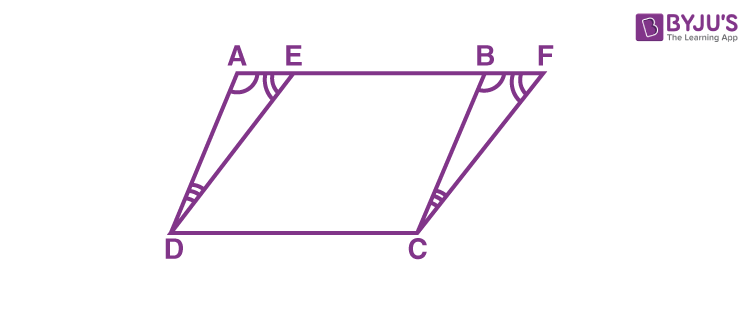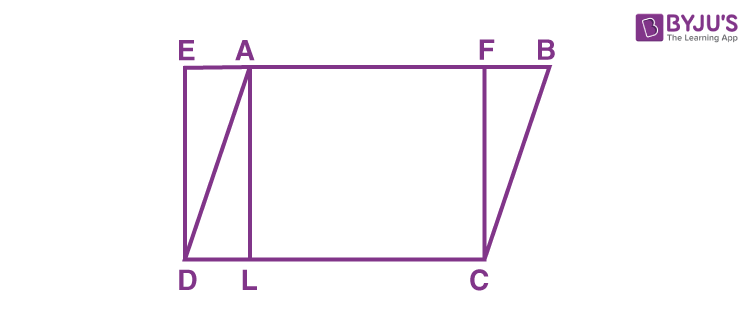# Parallelograms on the Same Base and Between the Same Parallels

Parallelograms on the same base and between the same parallels are explained here in this article with the help of the theorem.

## Theorem:

A parallelogram on the same base and between the same parallels are equal in area.

## Proof:Consider two parallelograms ABCD and EFCD on the same base DC and the same parallels AF and DC, as shown in the figure.

To prove: ar (ABCD) = ar (EFCD)

It means that we need to prove the area of parallelogram ABCD is equal to the area of a parallelogram EFCD.

Consider the triangles ADE and BCF,

∠ DAE = ∠ CBF [Corresponding angles from AD || BC and transversal AF] …(1)

∠ AED = ∠ BFC [Corresponding angles from ED || FC and transversal AF] …(2)

By using the angle sum property of a triangle, we can write:

∠ ADE = ∠ BCF ….(3)

Also, from the opposite sides of a parallelogram ABCD, we can write:

Using the equations (1), (3), and (4) and by Angle-Side-Angle (ASA) rule,

Therefore, the area of triangle ADE is equal to the area of a triangle BCF. (As congruent figures have equal area)

(i.e) ar (ADE) = ar (BCF) …(5)

Thus, the ar (ABCD) = ar (ADE) + ar (EDCB)

ar (ABCD) = ar (BCF) + ar (EDCB) (using equation (5))

ar (ABCD) = ar (EFCD)

Thus, the area of parallelogram ABCD is equal to the area of parallelogram EFCD.

Hence, a parallelogram on the same base and between the same parallels are equal in area, is proved.

### Example

Now, let us solve the below problem with the help of the theorem.

Question:

Consider a parallelogram ABCD and EFCD is a rectangle. Also, AL is perpendicular to DC.Prove that:

1. ar (ABCD) = ar (EFCD)
2. ar (ABCD) = DC × AL

Solution:

Given that ABCD is a parallelogram, and EFCD is a rectangle.

To prove : ar (ABCD) = ar (EFCD)

A rectangle is also a parallelogram, and by using the theorem “parallelogram on the same base and between the same parallels are equal in the area”, we can write

ar (ABCD) = ar (EFCD)

Hence proved.

To prove: ar (ABCD) = DC × AL

By using ar (ABCD) = ar (EFCD)

ar (ABCD) = DC × FC (As, the area of a rectangle = Length × Breadth) …(1)

Also given that AL ⊥ DC, we can say AFCL is also a rectangle.

Therefore, AL = FC …(2)

From (1) and (2), we can get

ar (ABCD) = DC × AL

Hence, proved.

### Practice Problems

Solve the following problems:

1. The point P and Q lie on the sides DC and AD respectively of a parallelogram ABCD. Prove that ar (APB) = ar (BQC)
2. In the figure given below, PQRS and ABRS are parallelograms. “X” is the point on the side BR.Prove that:

(1) ar (PQRS) = ar (ABRS)

(2) ar (AX S) = ½ ar (PQRS)

Stay tuned with BYJU’S – The Learning App and learn all Maths-related topics easily by exploring more videos.